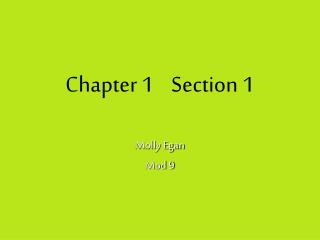DownloadDownload PresentationChapter 1 Section 1

# Chapter 1 Section 1

Download Presentation## Chapter 1 Section 1

- - - - - - - - - - - - - - - - - - - - - - - - - - - E N D - - - - - - - - - - - - - - - - - - - - - - - - - - -
##### Presentation Transcript

1. Chapter 1 Section 1 Molly Egan Mod 9

2. PointsPointsPointsPoints A B Points are represented by dots. The names of the points are A,B,C, and D. Capital letters are used to name points. A point is not a think, it is actually a location. A point has no size and no thickness. C D

3. Lines Listen to The Line Begins to Blur: Nine Inch Nails l E B m D F E line m A line can be named by any two points on it. This is why the line has a variety of names. line BD, BC, CD, CB, DB, or DC line l, EF, or FE Lines extend infinitely in two directions. The arrows at the ends of the line show its extension.

4. Line Segments Line segments and lines are both made up of points and straight. However, segments have a definite beginning and end. S Prolly the most boring video ever, but it explains how to name leg segments correctly. P R X Q RS orSR Vertical seg: PX or XP Horizontal seg: XQ or QX A segment is named by its endpoints.

5. Listen to Spoon: My Mathematical Mind Rays Just like segments and lines, rays are straight and made of points. A ray is different because it starts at an endpoint and then extends infinitely in one direction. B E D C Y A X CD or CE Endpoint must be given first, but then any other point on the ray may be used to name it. YX AB

6. Angles An angle is two rays with a common endpoint. The point is called the vertex of the angle and the rays are called the sides. A Y P B C 3 1 2 O R X 3 BAC CAB A This angle cannot be named angle O because that could refer to two different angles. 1 can be POY or YOP 80’s music video “Angle Dance” 2can be XOY, ROY, YOX, orYOR D D

7. TRIANGLES TRIANGLES A TRIANGLES TRIANGLES TRIANGLES TRIANGLES B C TRIANGLES A triangle has three segments as its sides. Even though there are no arrows in the diagram, you can still say LB. A triangle has three sides and three angles. It is the union of three segments. A vertex is the intersection of any two sides. TRIANGLES TRIANGLES TRIANGLES TRIANGLES

8. Sample Problems Problem 1: a. How many lines are shown? (Imagine there’s arrows in the diagram) b. Name these lines. c. Where do AC and DE intersect? d. Where does AC intersect BC? e. What is the union of BA and BD? A E B m D C

9. Sample Problems Problem 2: a. Name the ray that has endpoint A and goes in the direction of C b. Name the segment joining A and B B C A

10. Sample Problems Problem 1 and 2 Answers: 1. a. 2 b. Line m, DB, DE, BD, EB, EB, or ED; AB, AC, BA, BC,CA, or CB. c. B d. AC e. L ABD 2. a. AB or AC b. AB or BA

11. Problem 3 and 4: 3. Draw a diagram in which the intersection of AB with CA is AC. 4. Draw a diagram in which Sample Problems

12. Answer problem 3: Answer problem 4: Sample Problems A B C A E F D B C

13. MoreSampleProblems 5. Can this ray be called BA? A B 6. Fill in the Blank 7. A triangle is the ______ of three segments. 8. A segment has ____ endpoints. True or False 9. Rays extend in two directions 10. The simplest polygon is a triangle. 11. Points on a line have length and with. A B E C D

14. Answers 5. No, but it can be called AB. It can’t be BA because a ray’s name must always begin with it’s endpoint. 6. CB, L ACD, E, B 7. Union 8. 2 9. False, rays extend infinitely in only one direction. 10. True 11. False, points do not have a length or width. They are merely a location on a line.

15. Bibliography Rhoad, Richard, Milauskas, and Robert Whipple. Geometry for Enjoyment and Challenge. New Edition. Evanston, Illinois: McDougal Littell, 1991. "Basic Terms." Math League. August 2006. Math League Press. 30 May 2008 <http://www.mathleague.com/help/geometry/basicterms.htm>.